Function Repository Resource:

AlgebraicSubstitutionTiling

Return a substitution tiling

Contributed by: Ed Pegg Jr
 ResourceFunction["AlgebraicSubstitutionTiling"][tiling,steps] with the given tiling system specified by tiling, return the state after steps substitutions.

Details and Options

A third argument can specify alternate starting states or numeric approximations (faster).
The possible named values for tiling are: "PinwheelTiling", "DominoTiling", "TrominoTiling", "LTetrominoTiling", "PPentominoTiling", "Tritan3Tiling", "Tritan4Tiling", "TriangleDuo", "WaltonChair", "SqrtTwo", "SqrtTwo2", "Drafter3", "Drafter4", "HalfHex", "QuarterHex", "Kite", "SphinxTiling", "LimhexTiling", "AmmannA4Tiling", "AmmannChairTiling", "KitesandDartsTiling", "PenroseTriangleTiling", "PenroseRhombTiling", "BinaryTiling", "SqrtPhi", "SqrtPsi", "SqrtRho", "SqrtRho2", "SqrtRho3", "SqrtRho4", "TribonacciChordQuadrangle", "SqrtChi", "SqrtChi2", "SqrtChi3", "SqrtChi4", "QuarticPinwheel".

Examples

Basic Examples

Show a starting kite after two substitution steps with the Penrose kites and darts tiling system:

 In:=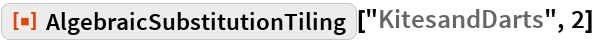Out=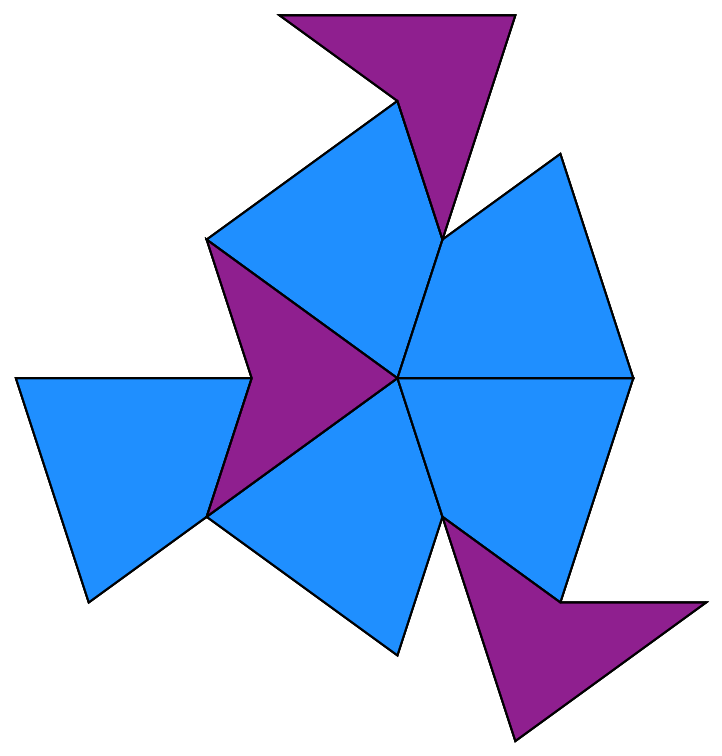With steps=0, the substitution tiling itself is shown, as with the Penrose kites and darts tiling system:

 In:=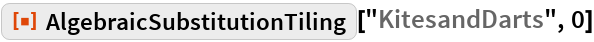Out=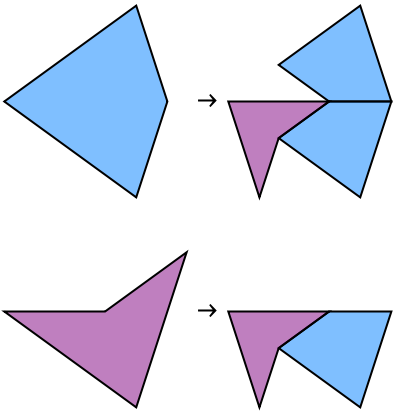Show the pinwheel tiling with an alternate start:

 In:=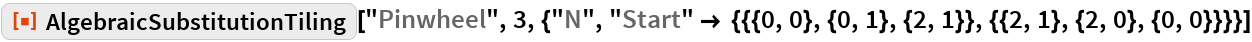Out=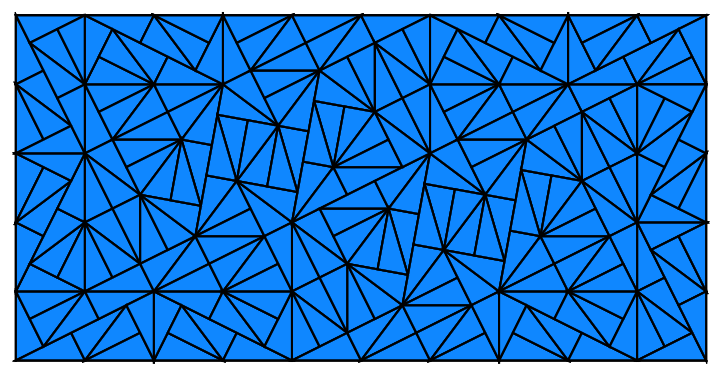Scope

Show the numerically approximated second step of all name-supported tilings:

 In:=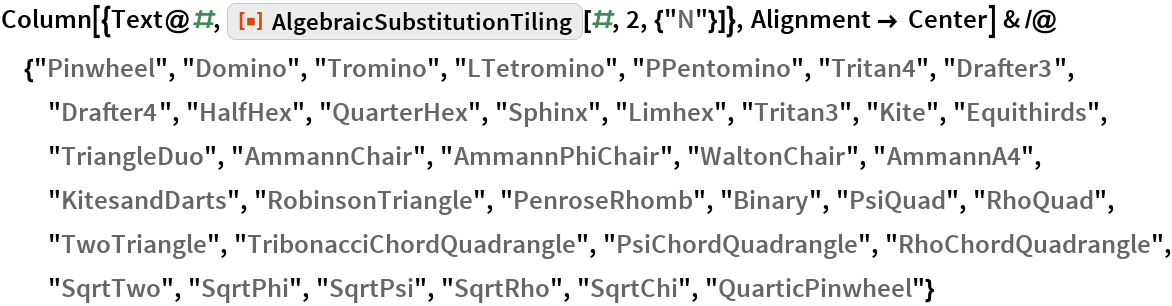Out=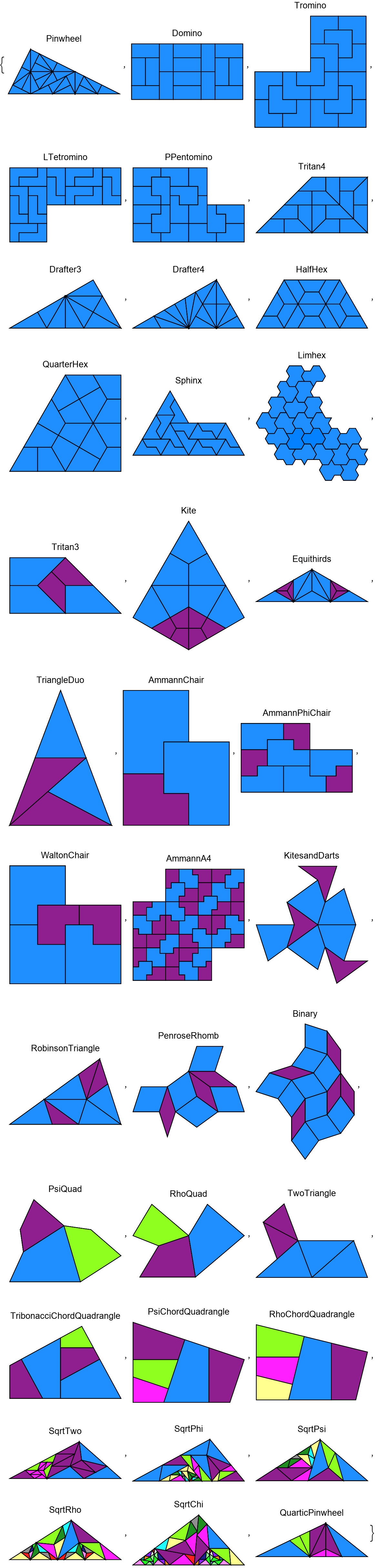Options

The Penrose kites&darts tiling system with the root, algebraic points in terms of that root, polygon substitutions and polygon types:

 In:=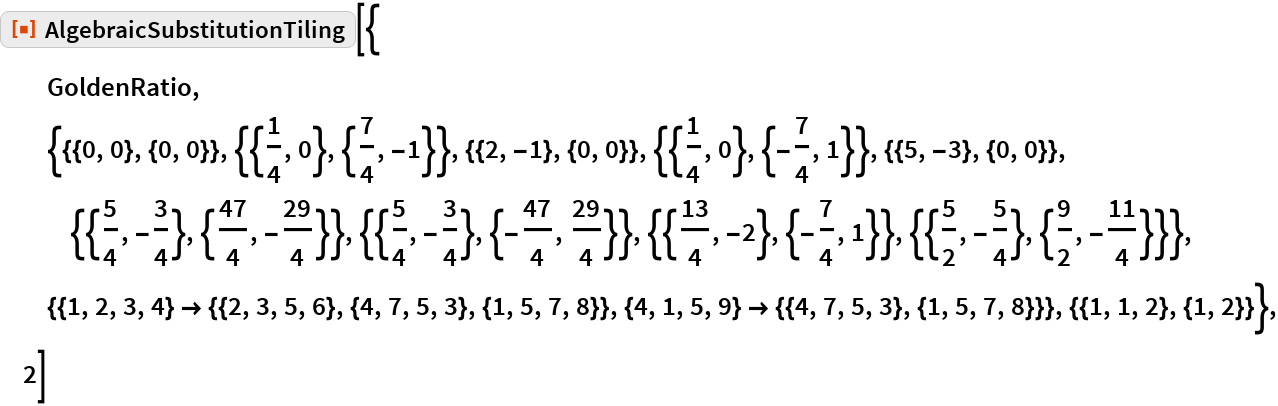Out=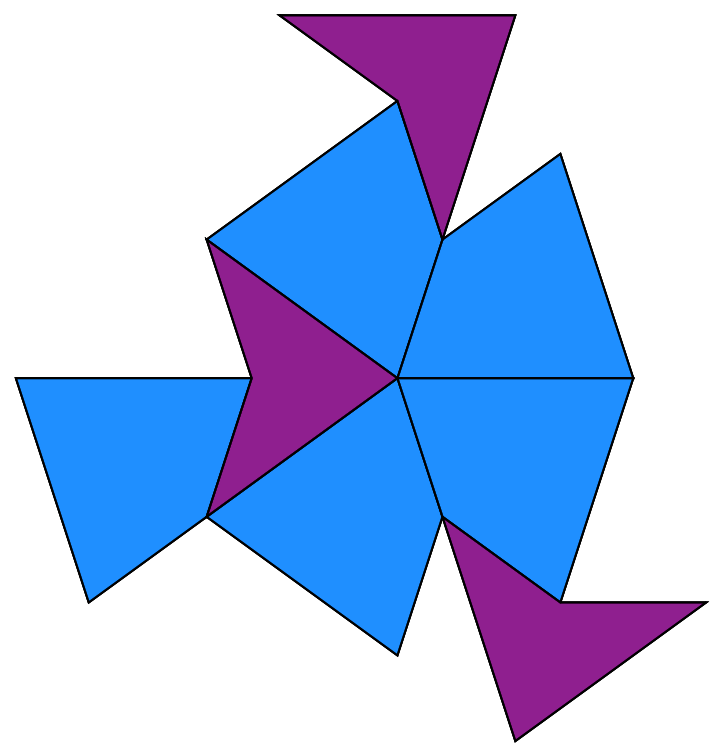A chord tiling with a plastic constant (ρ) root, algebraic points in terms of ρ, polygon substitutions and polygon types:

 In:=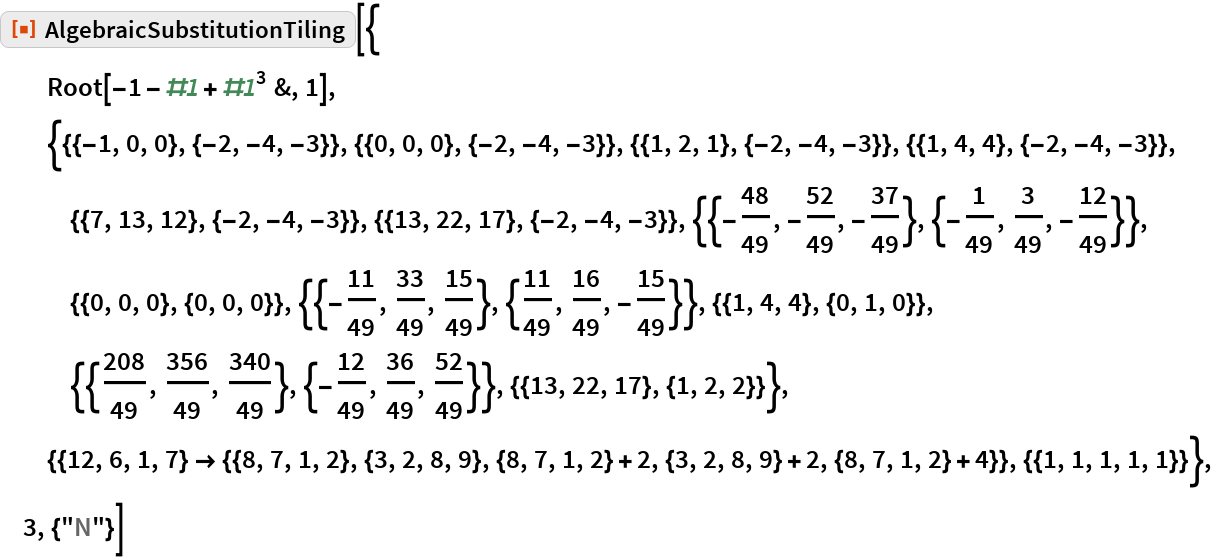Out=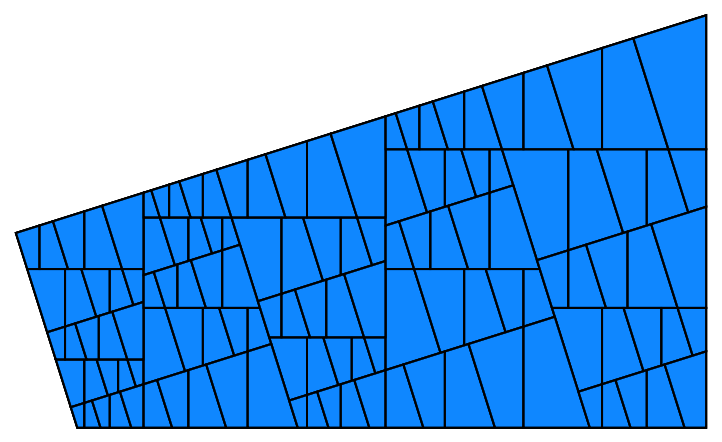Applications

Show polygons of every step as a layered graphic:

 In:=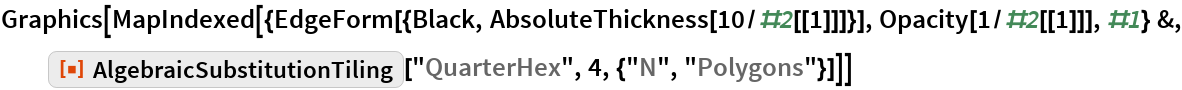Out=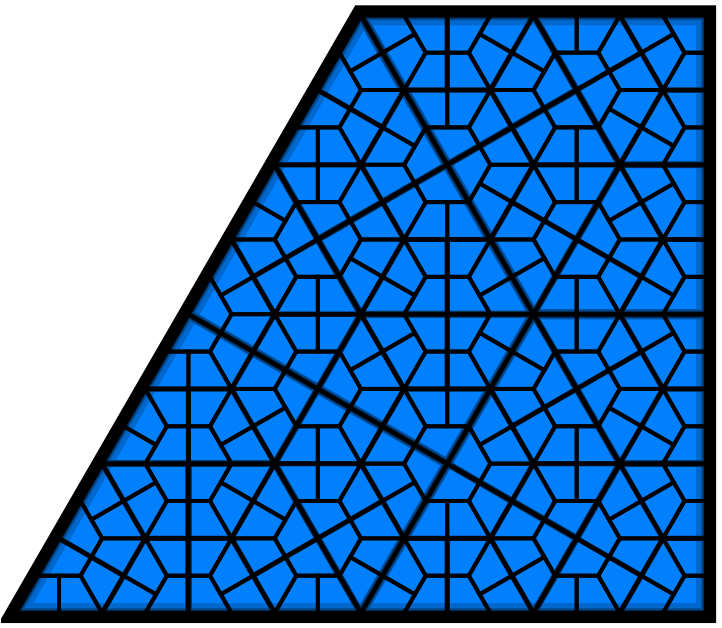Neat Examples

The psi-quad substitution tiling is based on the Narayana cow sequence constant, ψ≈1.465571231876768, also called the super-golden ratio, shown here with sixteen levels of substitution:

 In:=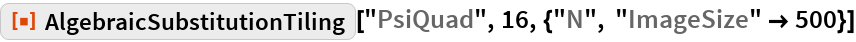Out=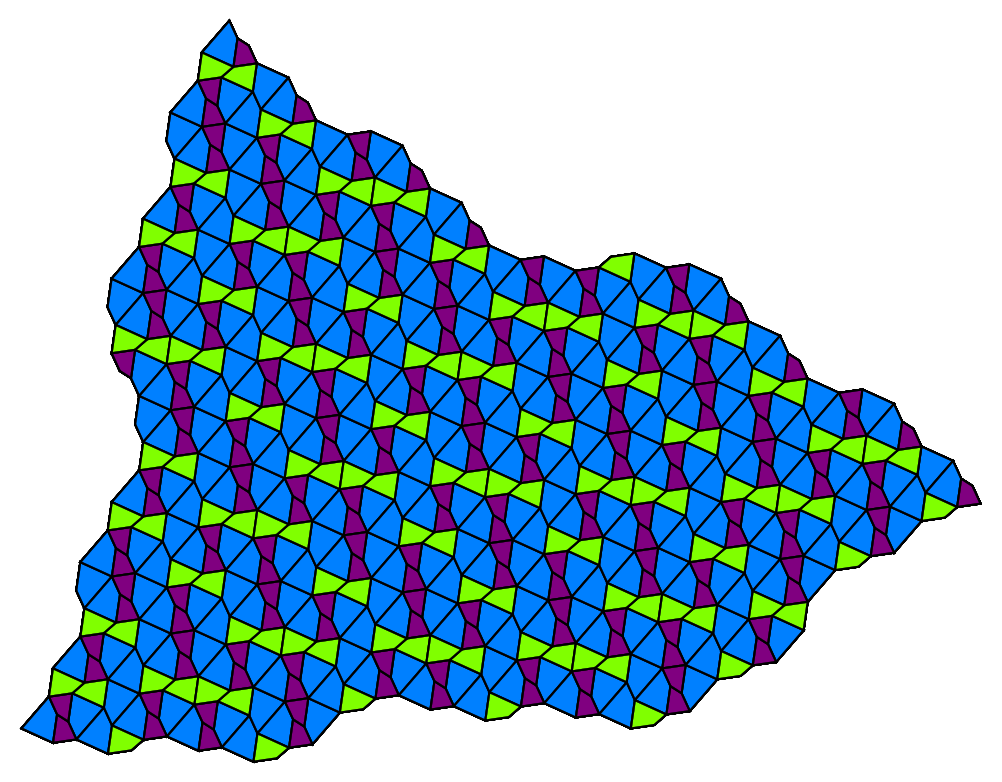The rho-quad substitution tiling is based on the plastic constant, ρ≈1.324717957244746, shown here with thirteen levels of substitution on top of the initial tile:

 In:=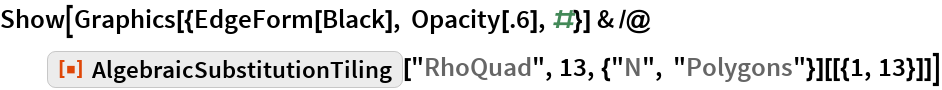Out=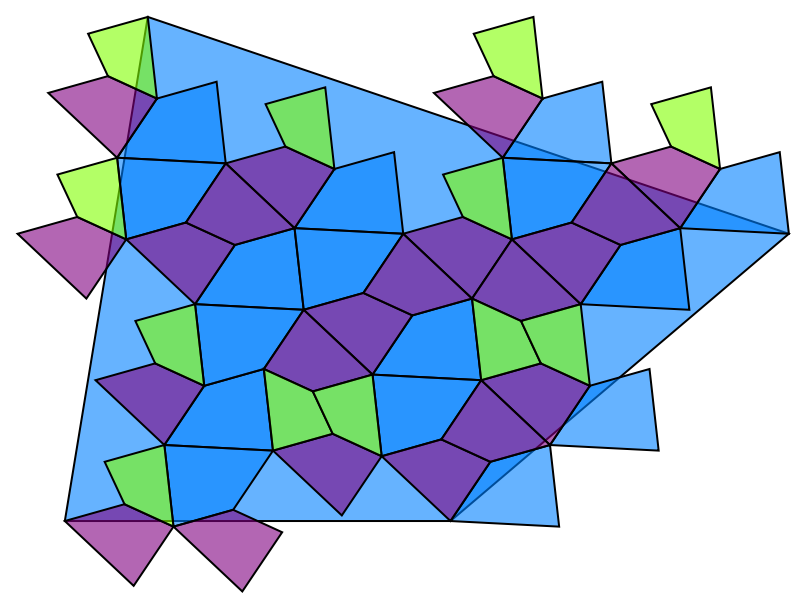Use numeric approximation to show and compare growth with alternate starting positions for the Penrose rhombs and the Godrèche-Lançon binary tiling systems:

 In:=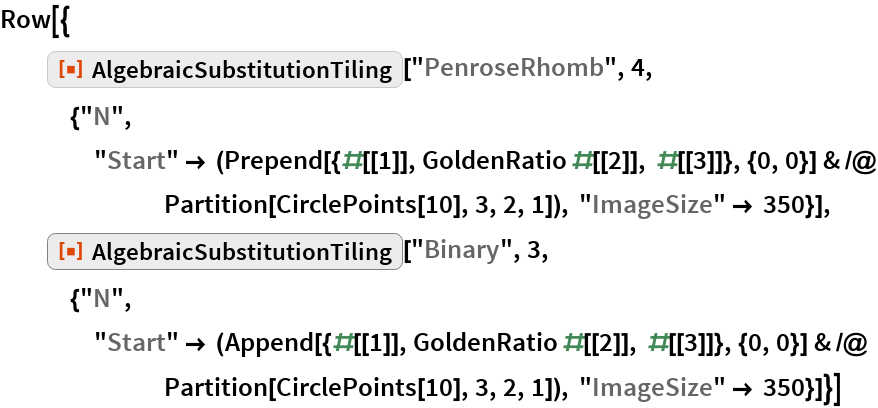Out=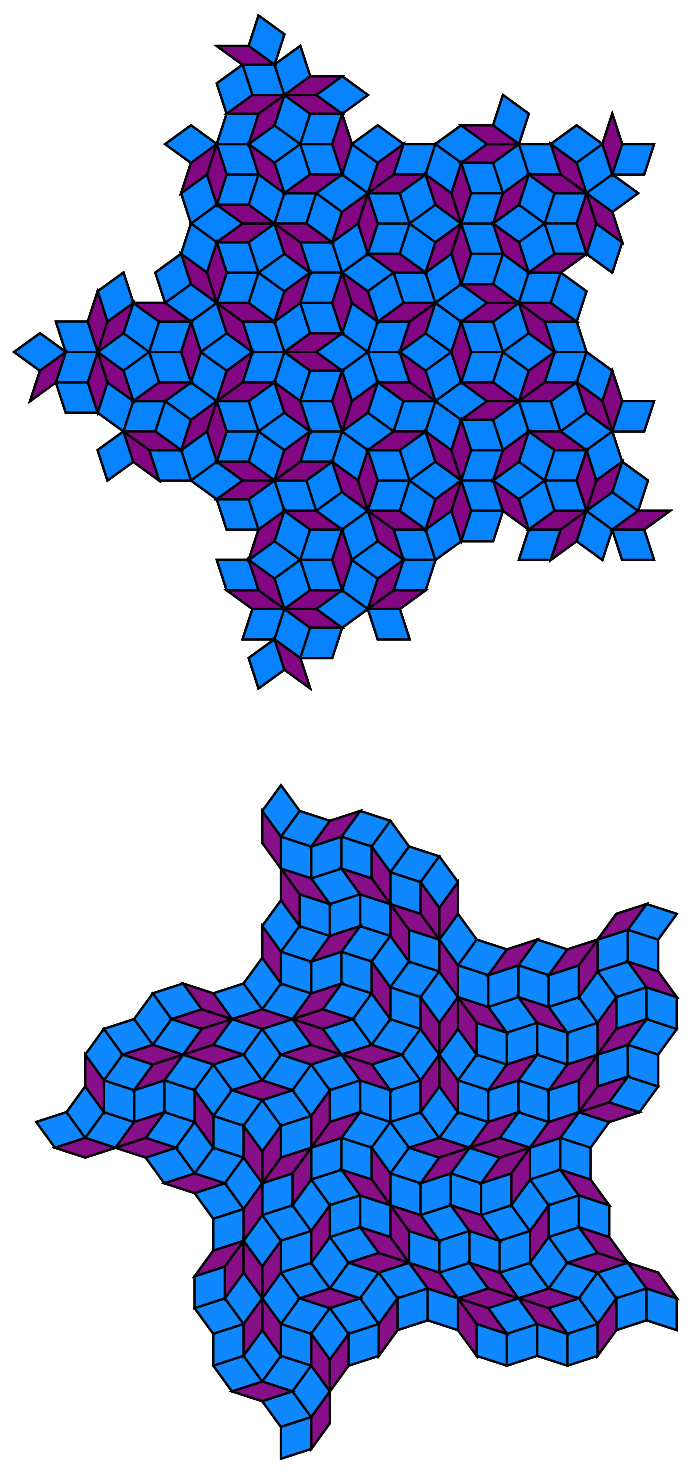Tilings from the blog article Shattering the Plane with Twelve New Substitution Tilings Using 2, φ, ψ, χ, ρ:

 In:=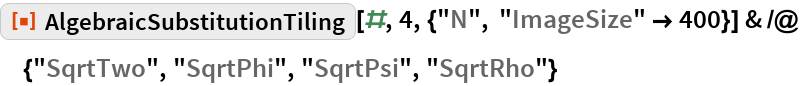Out=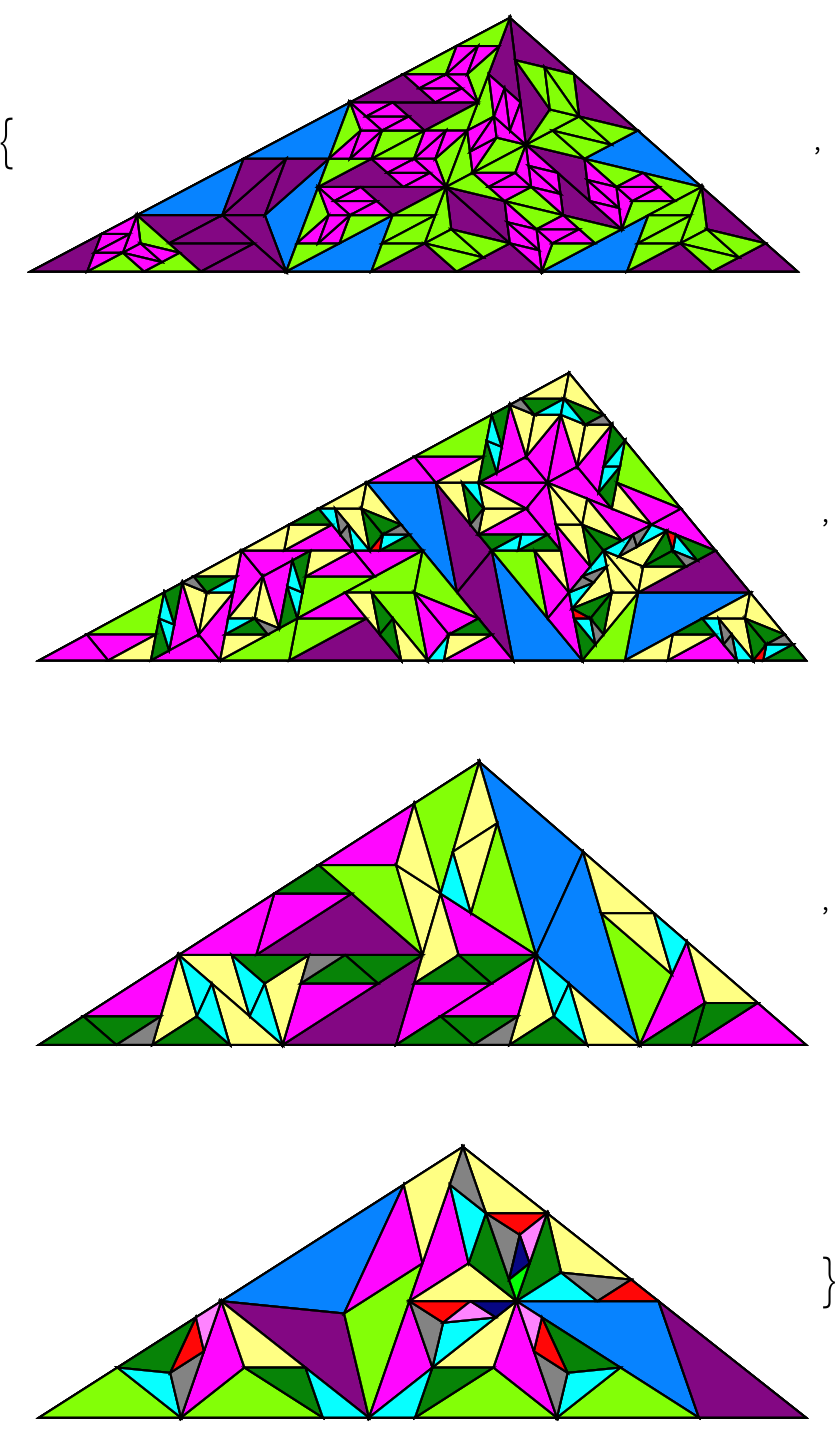One of many variants of a tiling based on a root of χ4-χ-1=0:

 In:=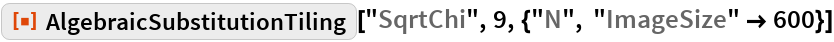Out=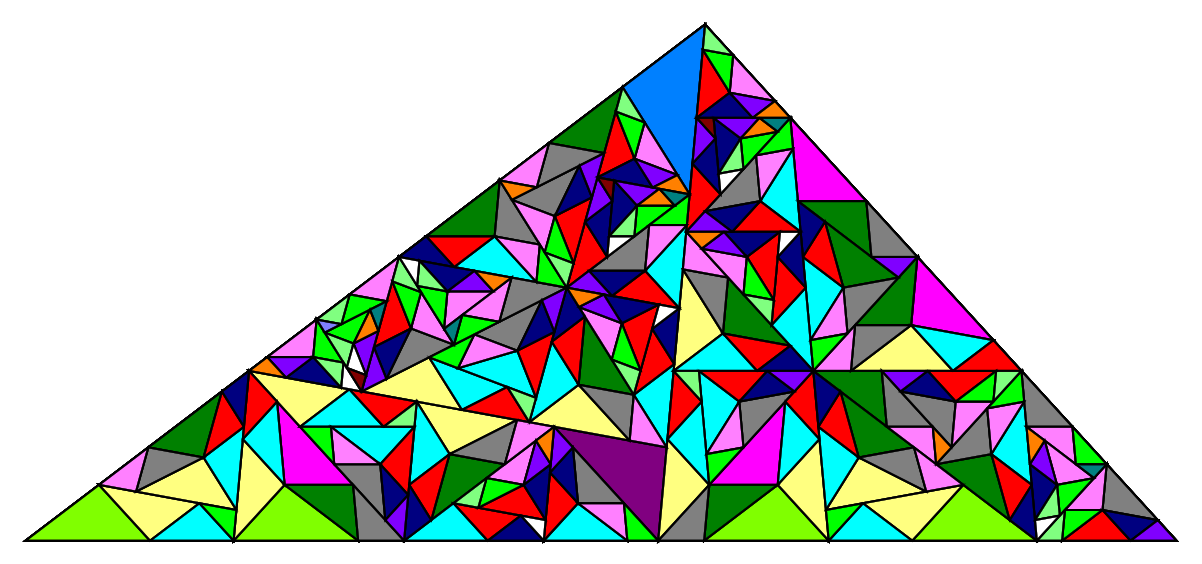Counts of the triangles of different sizes in the above tiling:

 In:=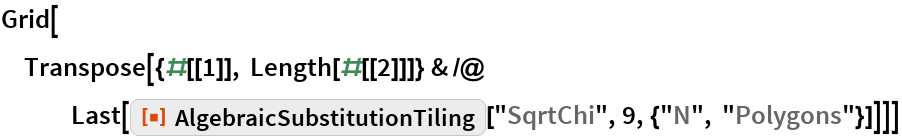Out=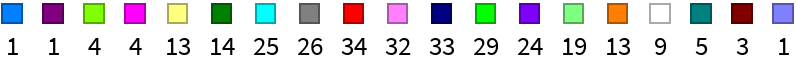Requirements

Wolfram Language 11.3 (March 2018) or above